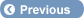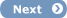ExpertRating HomeExpertRating BenefitsRecommend ExpertRatingSuggest More TestsPrivacy PolicyFAQLogin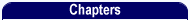JavaScript Tutorial - IndexJavaScript Tutorial - Introduction to JavaScriptJavaScript Tutorial - Basic JavaScript ConceptsJavaScript Tutorial - Working with Operators and VariablesJavaScript Tutorial - How to work with Loops and Functions?JavaScript Tutorial - How to work with Forms and Events?JavaScript Tutorial - How to work with Objects?JavaScript Tutorial - How to work with Browsers and Cookies?JavaScript Tutorial - More about ObjectsJavaScript Tutorial - How to validate Forms with JavaScript?JavaScript Tutorial - Advanced Techniques in JavaScript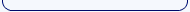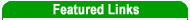Online Personal Trainer CertificationPersonal Trainer Certification (Spanish)Six Sigma CertificationFind a Personal TrainerProject Management CertificationSix Sigma CertificationSix Sigma Black Belt CertificationMaster Black Belt CertificationSix Sigma Green Belt CertificationLife Coach CertificationLean Management CertificationLean Six Sigma CertificationFirst Aid CertificationCPR CertificationYoga CertificationAerobics CertificationPilates CertificationBusiness Analyst CertificationSEO CertificationOnline Business Writing Course & CertificationMakeup Artist CertificationBaby Sitting Course & CertificationTime Management CertificationHealth Club Management CertificationMedical Coding CertificationMedical Billing CertificationBusiness Analysis CourseMaster Trainer CertificationTotal Quality Management CertificationKaizen CertificationCreative Writing CertificationEvent Management CertificationTest Search by Job TitleAdministrative Assistant CertificationVirtual Assistant Certification All Online Courses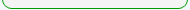Home > Courses, Tutorials & eBooks > JavaScript Tutorial > Working with Operators and Variables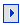## JavaScript Tutorial - Working with Operators and Variables

Page 2 of 3

Variable Scope

The scope of a variable defines the lifetime of that variable. All variables have scope. When a variable's lifetime comes into scope, the variable becomes accessible. Its value can be set or retrieved. When a variable's lifetime moves out of scope, that variable is no longer accessible. A variable can have either 'Local' or 'Global' scope.

When a function is defined, certain variables are used for storing values. Variables that only exist inside a function, are called Local variables.The values of local variables cannot be changed by other functions. Hence local variables have local scope. This results in easy code maintenance and is helpful if many programmers are working together on the same project. Variables that exist throughout the script are called Global variables. Their values can be accessed by any other function, thus they have global scope.

Operators

An operator transforms one or more values into a single resultant value. The values on which operator  is applied is referred as 'operand'. A combination of an operator and its operands is referred to as an expression. There are three types of operators : binary( it works on two operands), unary( it has only one operand) and ternary( it has three operands). Expressions are evaluated to determine the value of the expression. The most common operators are summarized below:

Arithmetic Operator

Arithmetic operators are also known as computational operators. They perform a mathematical function on a value or values and return a single value.

 Operator Description Example + Addition or Concatenation of two strings 6+7 = 13 ; or "Java"+"Script"=" JavaScript" - Subtraction or Unary negation 11-5 = 6;  or   -7 * Multiplication 12*12= 144 / Division 16/2 = 8 % Modulus ( Calculates the remainder) 16%3 = 1 ++ Increment x=2; x++; Result = 3 -- Decrement x=4; x--; Result = 3

Assignment Operator

The assignment operator is used to update the value of a variable. Usually assignment operators are combined with other operators to perform a computation. Assignment operators supported by JavaScript are :

 Operator Description Example = Assignment sign, assigns right variable to value to the left variable. x = y += Increments left variable by the value of the expression on the right. If used with strings, the value at right side is appended to the value of the variable on the left side. x += y; x = x + y -= Decrements left variable by the value of the expression on the right. x -= y; x = x-y *= Multiplies left variable by the value of the expression on the right. x *= y; x = x*y /= Divides left variable by the value of the expression on the right. x /= y; x = x/y %= Takes the modulus of the left variable using the value of the expression on its right. x %=y; x = x % y

Comparison Operator

Comparison operators are used to compare two values. Comparison operators supported by JavaScript are:

 Operator Description Example = = Equal- The equal to operator returns true if both operands are equal and false if not. It performs data type conversion too. x=5 ; y="5" ; x = =y Result : True = = = Strictly Equal- The strict equal to operator returns true if both operands are equal (and of the same type). It doesn't perform any data type conversion. x=5 ; y="5" ; x = = =y; Result : False != Not Equal- The not equal to operator returns true if both operands are not equal and returns false if they are equal. It also performs data type conversion. x = 5 ; y = 8; x != y; Result : True != = Strictly Not Equal- The strict not equal to operator returns true if both operands are not equal. It will not perform any data type conversion. x =5 ; y = "5" ; x!=y; Result : True < Less than- The less than operator produces the value true if the first operand is less than the second operand, and produces the value false if not. Comparison operators can work on string operands too. x = 5; y = 8; x < y; Result : True <= Less than or equal to- The less than or equal to operator produces true if the first operand is less than or equal to the second operand, and produces false if not. x = 5; y = 5; x <= y; Result : True > Greater than- The greater than operator produces the value true if the first operand is greater than the second operand, and produces false if not. x = 15; y = 8; x > y; Result : True >= Greater than or equal to- The greater than or equal operator produces true if the first operand is greater than or equal to the second operand, and produces false if not. x = 15 ; y = 15; x >= y; Result : True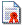ExpertRating JavaScript Certification - Get Certified Now!!